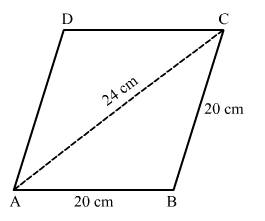# Find the area of a rhombus one side`
Question:

Find the area of a rhombus one side of which measures 20 cm and one of whose diagonals is 24 cm.

Solution:

It is given that,
The sides of rhombus = 20 cm.
One of the diagonal = 24 cm.In ∆ABC,
The sides of the triangle are of length 20 cm, 20 cm and 24 cm.
∴ Semi-perimeter of the triangle is

$s=\frac{20+20+24}{2}=\frac{64}{2}=32 \mathrm{~cm}$

∴ By Heron's formula,

Area of $\Delta A B C=\sqrt{s(s-a)(s-b)(s-c)}$

$=\sqrt{32(32-20)(32-20)(32-24)}$

$=\sqrt{32(12)(12)(8)}$

$=192 \mathrm{~cm}^{2} \quad \ldots(1)$

In ∆ACD,
The sides of the triangle are of length 20 cm, 20 cm and 24 cm.
∴ Semi-perimeter of the triangle is

$s=\frac{20+20+24}{2}=\frac{64}{2}=32 \mathrm{~cm}$

∴ By Heron's formula,

Area of $\Delta A C D=\sqrt{s(s-a)(s-b)(s-c)}$

$=\sqrt{32(32-20)(32-20)(32-24)}$

$=\sqrt{32(12)(12)(8)}$

$=192 \mathrm{~cm}^{2} \quad \ldots(2)$

∴ Area of the rhombus = Area of ∆ABC + Area of ∆ACD

= 192 + 192

= 384 cm2

Hence, the area of a rhombus is 384 cm2.